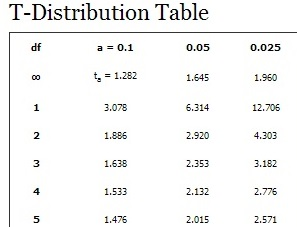# Degree of Freedom

## What are Degrees of Freedom?

Degrees of Freedom describes the number of independent ways a dynamic system can move without impeding any of the constraints placed upon it. Put simply, it is the number of values in a function that are free to vary. Often, Degrees of Freedom are defined as one less that the value of the sample size, as defined by (DF = N-1).

## Degrees of Freedom for Multiple Samples

Sometimes it is useful to calculate the Degrees of Freedom of multiple sample sets. For example, to find the Degrees of Freedom for two samples one needs to account for both samples in the formula. Therefore, the formula for Degrees of Freedom of two samples is defined as DF = (N1 + N2) - 2.Degrees of Freedom as displayed in a T-Distribution table
Source

### Degrees of Freedom and ANOVA

ANOVA tests compare multiple means to each other, rather than a single data set. Naturally, this produces multiple means, one for each set. There are actually two Degrees of Freedom in ANOVA, marked DF1 and DF2. For a two-group ANOVA, the Degrees of Freedom are defined by the formula (DF1 = N-1). However, for a three or more group ANOVA, the Degrees of Freedom product is the total number of observations in all cells, minus the degrees of freedom lost because all of the means are fixed. This concept is represented as (DF2 = N-K) where K is the number of cell means.

### Degrees of Freedom and Machine Learning

Neural networks use degrees of freedom to define the independent variables in a calculation. As a neural net is trained, its weights initially start out small and can become arbitrarily large. Accordingly, the number of degrees of freedom initially start out small and scale as well. As training progresses, the increase in the size of the class of functions that a neural net can approximate translates to an increase in the number of degrees of freedom.